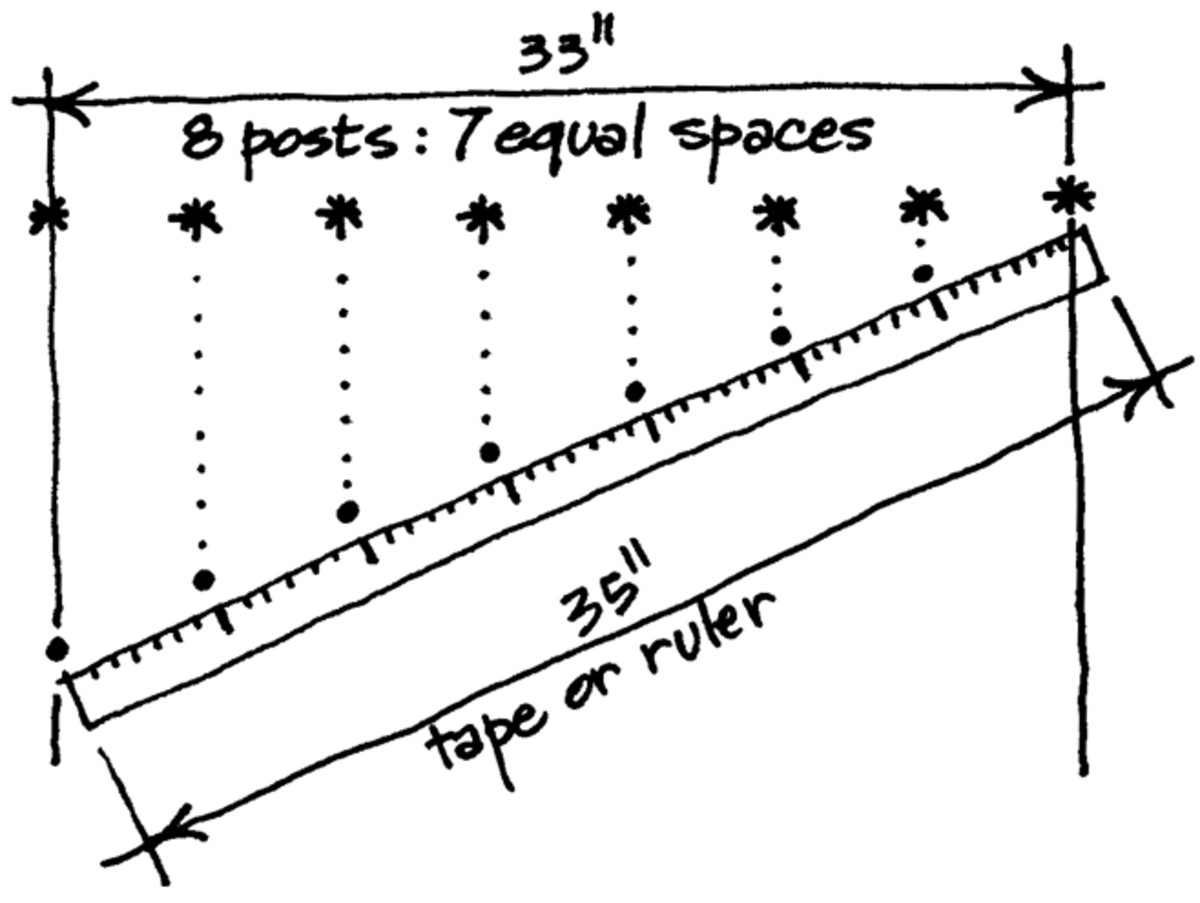# How to Easily Measure Equal SpacingsLearn the easiest method to measure equal spacings. Use the example in this image as you read.

## Calculating Even Spacing

Perhaps you've had fits trying to properly position a regular floor tile pattern in your rec room. Or maybe you struggled for hours to space those pictures down the wall in the hall.

Well, here are some simple instructions that can guide you to easily measure equal spacings for such items as railing posts, stairs, paving stones, floor or ceiling tiles, light fixtures, coat hooks, pictures, and so on—even if those equal spacings end up being some strange dimension, like 4 and 23/32".

Before we go through the steps for easily measuring equal spacings, we need to make sure you have the tools necessary for the job.

## Tools You'll Need to Evenly Space Objects

You will need the following tools in order to successfully execute this job:

• A ruler or tape measure a bit longer than the overall distance you are trying to subdivide into equal spacings.
• A pencil, marker, chalk, tape, stakes, or other means of marking off your equal spacings as you establish them.
• A straightedge will also come in handy.

## Step-By-Step Instructions

1. First, determine how many equal spacings you wish to measure. (As our example illustrated above, let's assume that we wish to place 8 equally spaced railing posts, with a total of 7 equal spaces between them, so that the line of railing posts runs a total distance of 33 inches along a single straight line.)
2. On your ruler or tape measure find and make note of a distance slightly greater than your overall running distance, that is also an even multiple of your number of equal spaces. (In our example above, we will choose to mark 35 inches, which is slightly greater than the overall running distance of 33 inches, and is also an even multiple of 7 equal spaces, because 7 x 5 = 35.)
3. Place the marked ruler or tape measure in a taut straight line along the overall distance or gap to be spanned by the equal spacings.
4. Keeping the ruler or tape measure in a taut straight line, adjust the angle of that line of the ruler or tape measure until your marked or noted endpoints exactly align with the endpoints of your overall distance or gap to be spanned. (As shown in our example above, the ruler or tape measure would run at an angle to our eventual line of railing posts, so that the point marked '0 inches' aligns with one end of our spanned distance, and the point marked '35 inches' aligns with the other end of our spanned distance.)
5. Now, simply mark the correct number of evenly spaced increments along the angled ruler or tape measure. (In our example, to mark each of the 7 equal space increments — since 7 x 5 = 35 — we would mark at 5 inch intervals along the ruler or tape measure, at '0 inches', '5 inches', '10 inches', '15 inches', '20 inches', '25 inches', '30 inches' and '35 inches'. This would give us a total of 8 large dots positioned at 7 exactly equal spacings.)
6. Finally, all we need to do is extend the dotted lines shown, to shift our 8 large dots to the line of our railing posts. We now have 8 large stars or asterisks positioned at 7 exactly equal spacings, and these are the final locations of our new railing posts.

## Final Note

By the way, in our example in the top image, those new railing posts will end up being spaced — not at 4 and 23/32" apart, as I hinted at the beginning — but in fact at 4 and 22.857/32" apart, which is an exceedingly difficult measurement to make by any other means!

## Calculating Equal Distance Tutorial

Question: I have 12 coat hooks for a 92" board. How much space should be between each hook?

Answer: If you want clearance for coats on the last hot on each end, then you need to create a 'half-space' at each end. So — 92" divided by 12 gives 7-2/3" (roughly 7-5/8") for each space between hooks. For 12 hooks, you need 11 spaces. 11 spaces x 7-5/8" = 77-55/8" or 83-7/8". 92" minus 83-7/8" leaves just about 8", to be split in half, with each half at each end of the row of hooks. If you want to keep things fairly simple: Start Hook #1 four inches from one end, then place Hook #2 7-5/8" farther, then place Hook #3 7-5/8" farther again, then place Hook #4 7-5/8" farther yet, and so on. Hook #12 should end up just about 4-1/8" from the end. Voila! You will have 12 hooks evenly spaced.

Question: How far apart would I space three objects in a 58.5-inch area?

Answer: If you want all three objects spaced equally across a 58.5" space, then you would create four 'gaps' between the objects, and at each end of the overall space of 14.625". If you want the three items to take up the entire 58.5" space, with one object at each extreme end, and one in the middle, then you actually have only two 'gaps' at 29.25" each.

Question: How far apart would I space three equal openings in a 47-inch opening using 3/4-inch thick shelves?

Answer: If there will be a shelf at the top, plus one at the bottom, plus two intermediate shelves, they would total 4 x 3/4" in thickness = 3". If you then subtract those 3" from the overall opening of 47", you are left with 44". The divide 44" by 3 openings = 14-2/3" for each opening, or just about 14 - 11/16". If you only have a shelf at the bottom, but not at the top, your 3 equal openings should be about 14 - 7/8".